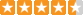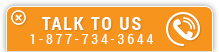# Basic Direct Current (DC) Theory

Course Number: E-4011
Credit: 4 PDH
Subject Matter Expert: A. Bhatia, Mechanical Engineer
Price: \$119.80 Purchase using Reward Tokens. Details20 reviews
Overview

#### In Basic Direct Current (DC) Theory , you'll learn ...

• Basic DC circuit calculations
• The polarities and circuit direction associated with DC circuits
• How to apply the laws governing DC circuits to DC circuit analysis
• The various effects of DC circuit faults

#### OverviewPreview a portion of this course before purchasing it.

Credit: 4 PDH

Length: 65 pages

Direct current or DC electricity is the continuous movement of electrons from an area of negative (-) charges to an area of positive (+) charges. A DC circuit is necessary to allow the current or stream of electrons to flow. Such a circuit consists of a source of electrical energy (such as a battery) and a conducting wire running from the positive end of the source to the negative terminal. DC circuits may be in series, parallel, or a combination. Understanding DC circuits is important for learning about the more complex AC circuits.

This course introduces the basic electricity and electrical circuit concepts associated with direct current. Topics include calculation of current, voltage, resistance, and power in series, parallel, and combination circuits with direct current power sources.

#### Specific Knowledge or Skill Obtained

This course teaches the following specific knowledge and skills:

• How DC voltage is produced and what an electric circuit consists of
• What a rectifier is and how half-wave bridge rectifiers differ from full-wave bridge rectifiers
• What the difference is between a schematic diagram, one-line diagram, block diagram, and wiring diagram
• What resistivity is and how temperature affects the resistance of materials
• The difference between open circuit, close circuit, and short circuit
• The identifying characteristics of a series, parallel, or combination circuit
• How to calculate the current and voltage drop in series, parallel, or combination circuits
• How to calculate the equivalent resistance for a series, parallel, or combination circuit
• What is meant by the terms voltage divider and current division
• How electron flow theory differs from conventional current theory

#### Certificate of Completion

You will be able to immediately print a certificate of completion after passing a multiple-choice quiz consisting of 20 questions. PDH credits are not awarded until the course is completed and quiz is passed.

Board Acceptance
 This course is applicable to professional engineers in: Alabama (P.E.) Alaska (P.E.) Arkansas (P.E.) Delaware (P.E.) Florida (P.E. Area of Practice) Georgia (P.E.) Idaho (P.E.) Illinois (P.E.) Illinois (S.E.) Indiana (P.E.) Iowa (P.E.) Kansas (P.E.) Kentucky (P.E.) Louisiana (P.E.) Maine (P.E.) Maryland (P.E.) Michigan (P.E.) Minnesota (P.E.) Mississippi (P.E.) Missouri (P.E.) Montana (P.E.) Nebraska (P.E.) Nevada (P.E.) New Hampshire (P.E.) New Jersey (P.E.) New Mexico (P.E.) New York (P.E.) North Carolina (P.E.) North Dakota (P.E.) Ohio (P.E. Self-Paced) Oklahoma (P.E.) Oregon (P.E.) Pennsylvania (P.E.) South Carolina (P.E.) South Dakota (P.E.) Tennessee (P.E.) Texas (P.E.) Utah (P.E.) Vermont (P.E.) Virginia (P.E.) West Virginia (P.E.) Wisconsin (P.E.) Wyoming (P.E.)
Reviews (20)
More DetailsPreview a portion of this course before purchasing it.

Credit: 4 PDH

Length: 65 pages